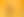Let’s Experiment: Discovering Area and Volume Formulas

# Stages1. Quadrilaterals: Investigating Areas of Trapeziums

EXPLORE

Suggested Learning Intentions

• To investigate the area formulas of trapeziums

Sample Success Criteria

• I can describe the similarities and differences between a parallelogram and trapezium
• I can develop the formula for calculating the area of trapeziums
• I can demonstrate and justify my thinking using a range of manipulatives
EXPLORE

2. Quadrilaterals: Investigating areas of Kites and Rhombuses

EXPLORE

Suggested Learning Intentions

• To investigate the area formulas of rhombuses and kites

Sample Success Criteria

• I can describe the similarities and differences between rectangles, parallelograms, kites and rhombuses
• I can develop the formula for calculating the area of kites and rhombuses
• I can use a range of manipulatives to model and explain my thinking
EXPLORE

3. Circles: Establishing Pi

EXPLORE

Suggested Learning Intentions

• To investigate the ratio of circumference to diameter

Sample Success Criteria

• I can describe how the radius, diameter and circumference of a circle are related
• I can demonstrate and justify my thinking using a range of manipulatives
• I can interpret the formula for the circumference of circles
EXPLORE

4. Circles: Establishing Area Formula

EXPLORE

Suggested Learning Intentions

• To visualise and evaluate how the area of a circle is determined

Sample Success Criteria

• I can explain the strategies used to develop the area formula for circles
• I can demonstrate my understanding of how the formula for area of circles is derived using a range of manipulatives
EXPLORE

5. Prisms: Establishing Volume Formulas

EXPLORE

Suggested Learning Intentions

• To build our understanding of the relationship between surface area and volume
• To develop the formula to find the volume of prisms

Sample Success Criteria

• I can identify the surface area of a prism
• I can describe the connection between surface area and volume
• I can describe the formula for calculating volume of prisms and explain why this is true for all prisms
• I can make connections between the area formula and volume formula
• I can demonstrate and justify my thinking using a range of manipulatives
EXPLORE